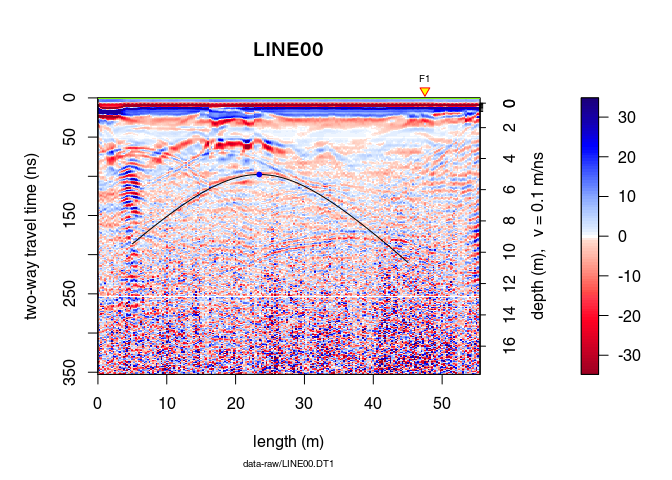# Hyperbola fitting

Note:

• This R-package is still in development, and therefore some of the functions may change in a near future.
• If you have any questions, comments or suggestions, feel free to contact me (in english, french or german): emanuel.huber@pm.me.

# Objectives of this tutorial

• Learn how to fit manually a hyperbola to GPR data

Note that his tutorial will not explain you the math/algorithms behind the different processing methods.

# Preliminary

## Install/load RGPR

# install "devtools" if not already done
if(!require("devtools")) install.packages("devtools")
devtools::install_github("emanuelhuber/RGPR")
library(RGPR)       # load RGPR in the current R session


## The GPR data

RPGR comes along with a GPR data called frenkeLine00. Because this name is long, we set x equal to frenkeLine00:

x <- frenkeLine00
plot(x)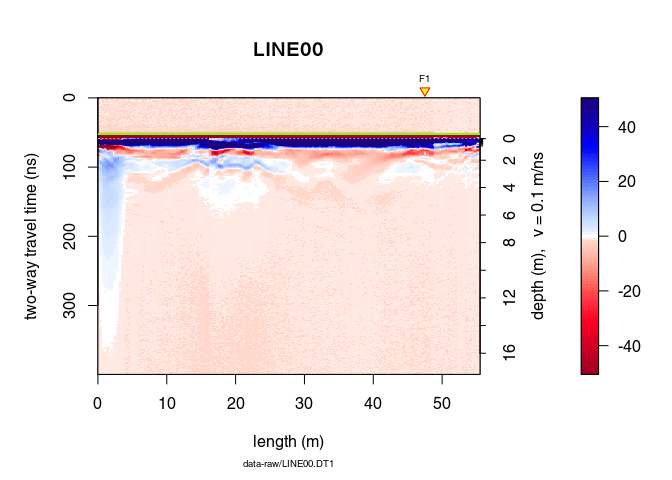## Basic processing

We apply some basic processing to the data with the pipe operator (%>%):

x <- x %>%  estimateTime0(w = 5, method = "MER", FUN = mean) %>%
time0Cor(method = "pchip")  %>%
dewow(type = "Gaussian", w = 5)  %>%
fFilter(f = c(200, 300), type = "low") %>%
gainSEC(a = 0.003, t0 = 50)

plot(x)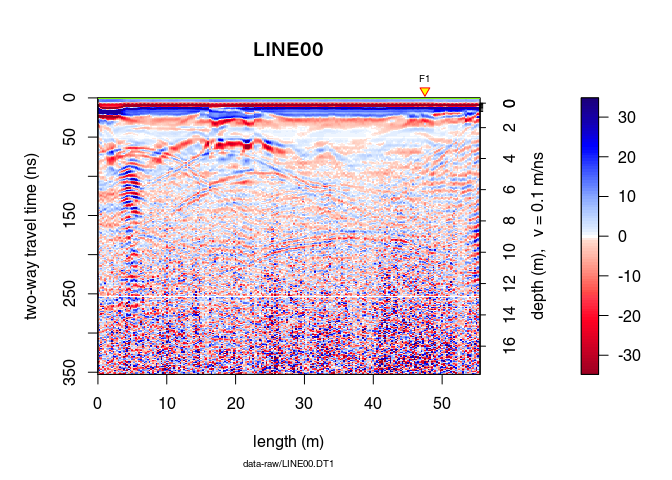# Hyperbola fitting

## Interactive point selection and hyperbola fitting

Select points interactively on the plot with

xy <- locator(type = "l")

## $x ##  11.8 15.0 17.7 20.3 24.4 27.4 30.9 35.2 ## ##$y
##  142.2 119.8 107.7  99.5  97.5 105.6 120.9 138.1


Fit the corresponding hyperbola with the function hyperbolaFit():

hyp <- hyperbolaFit(xy)


hyperbolaFit() returns:

• the hyperbola vertex: c(hyp$x0, hyp$t0)
• the estimated root-mean-square velocity: hyp$vrms • the estimated depth of the hyperbola vertex (computed using the root-mean-square velocity): hyp$z0
• the input points for the fitting: hyp$xand hyp$y
• the output of the regression fit (see R function lm()): hyp$reg ## Plot the fitted hyperbola • Plot the hyperbola for x-position ranging from 5 to 50: plot(x) points(xy, pch = 20, col = "blue") hyperbolaPlot(hyp, x = seq(5, 50, by = 0.01), col = "red", lwd = 2)• Plot the hyperbola without defining its x-position (in this case the hyperbola is displayed over the entire plot) plot(x) points(xy, pch = 20, col = "blue") hyperbolaPlot(hyp, col = "red", lwd = 2)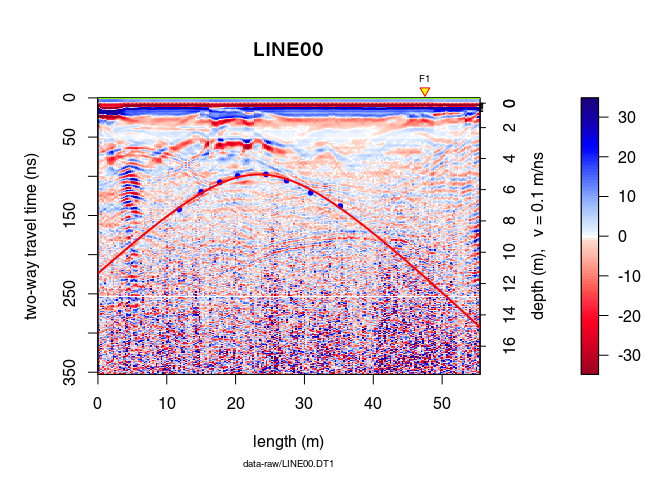• Define the hyperbola range with xlim and add some annotations: plot(x) points(xy, pch = 20, col = "blue") hyperbolaPlot(hyp, col = "red", lwd = 2, xlim = c(10, 40), ann = TRUE)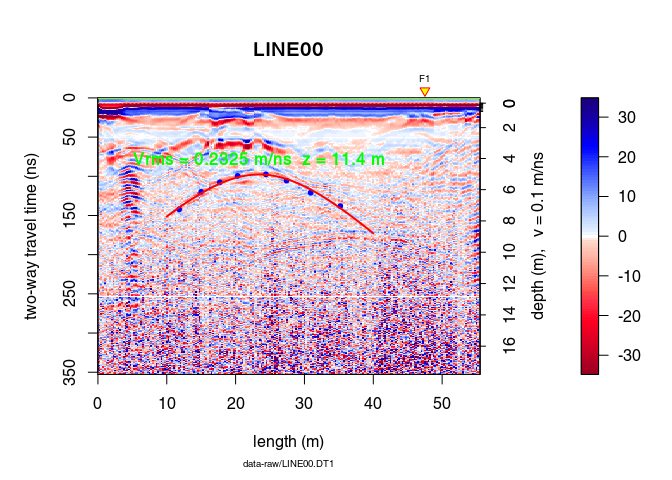## Plot an hyperbola defined by its vertex position and the root-mean-square velocity Define the hyperbola parameters hyp2 <- list(x0 = hyp$x0, t0 = hyp$t0, vrms = hyp$vrms)


Plot the hyperbola

plot(x)
points(hyp$x0, hyp$t0, pch = 20, col = "red", cex = 1.3)
hyperbolaPlot(hyp2, col = "blue", lwd = 2, ann = TRUE)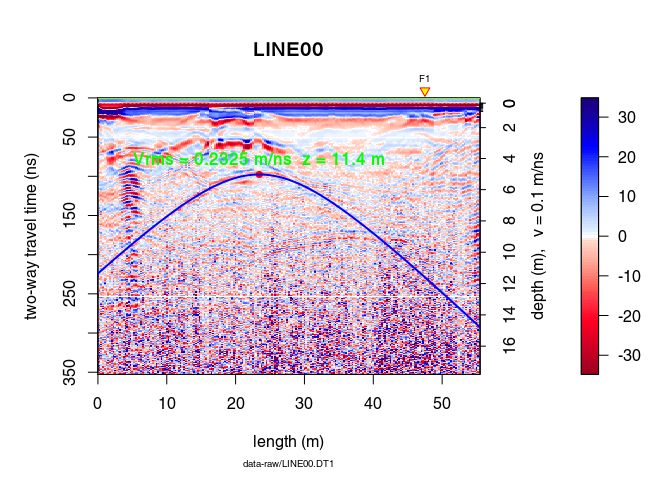## Simulate an hyperbola

• Using the output of hyperbolaFit():

xv <- seq(5, 45, by = 0.1)
y <- hyperbolaSim(xv, hyp)
plot(x)
points(hyp$x0, hyp$t0, pch = 20, col = "blue")
lines(xv, y)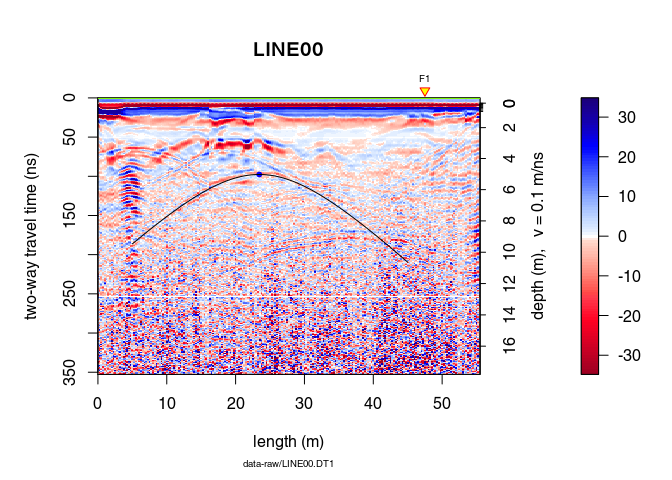• Defining its vertex position and the root-mean-square velocity:

hyp2 <- list(x0 = hyp$x0, t0 = hyp$t0, vrms = hyp$vrms) xv <- seq(5, 45, by = 0.1) y <- hyperbolaSim(xv, hyp2) plot(x) lines(xv, y) points(hyp$x0, hyp\$t0, pch = 20, col = "blue")